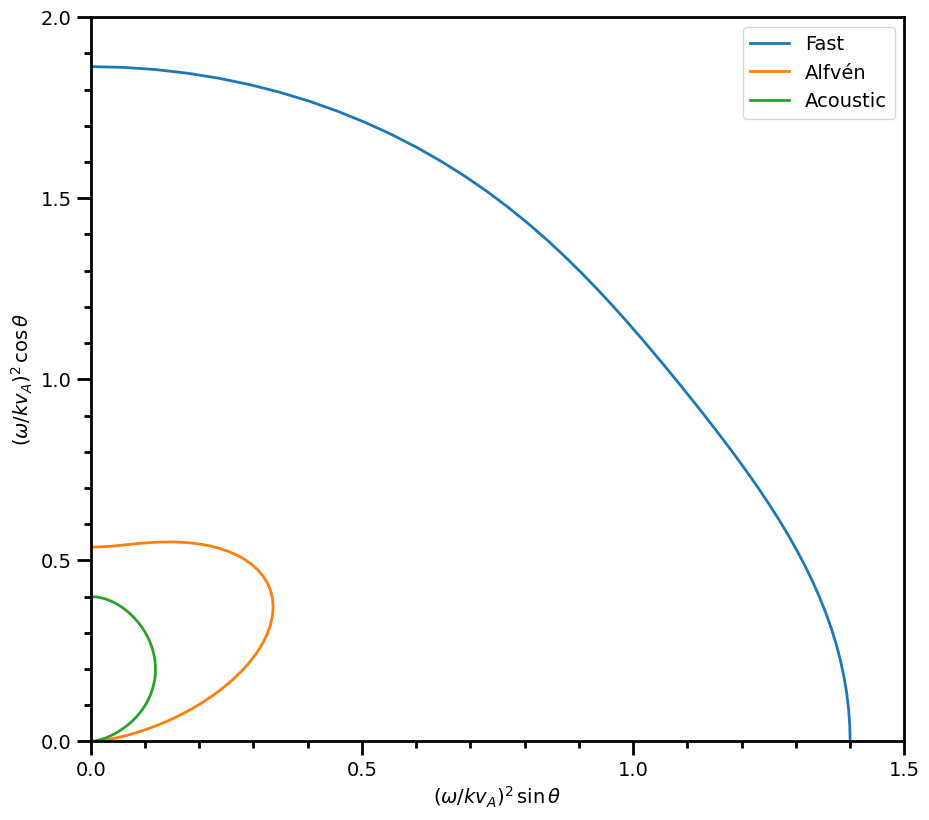# plasmapy.dispersion.analytical

The analytical subpackage contains functionality associated with analytical dispersion solutions.

## Sub-Packages & Modules

 two_fluid_ This module contains functionality for calculating various analytical solutions to the two fluid dispersion relation.

## Functions

 two_fluid(*, B, ion, k, n_i, T_e, T_i, theta) Using the solution provided by Bellan , calculate the analytical solution to the two fluid, low-frequency ($$\omega/kc \ll 1$$) dispersion relation presented by Stringer .

## Example Notebooks